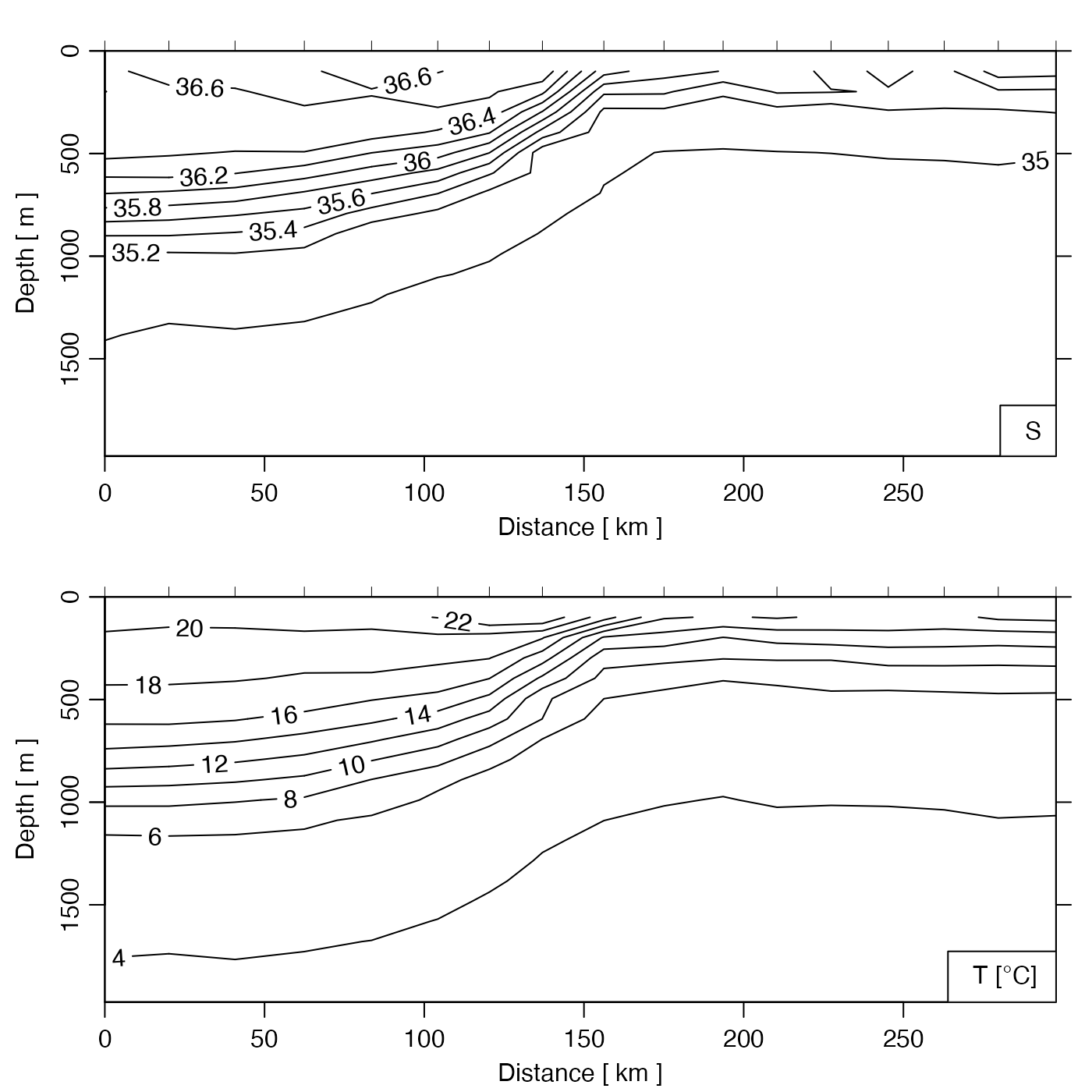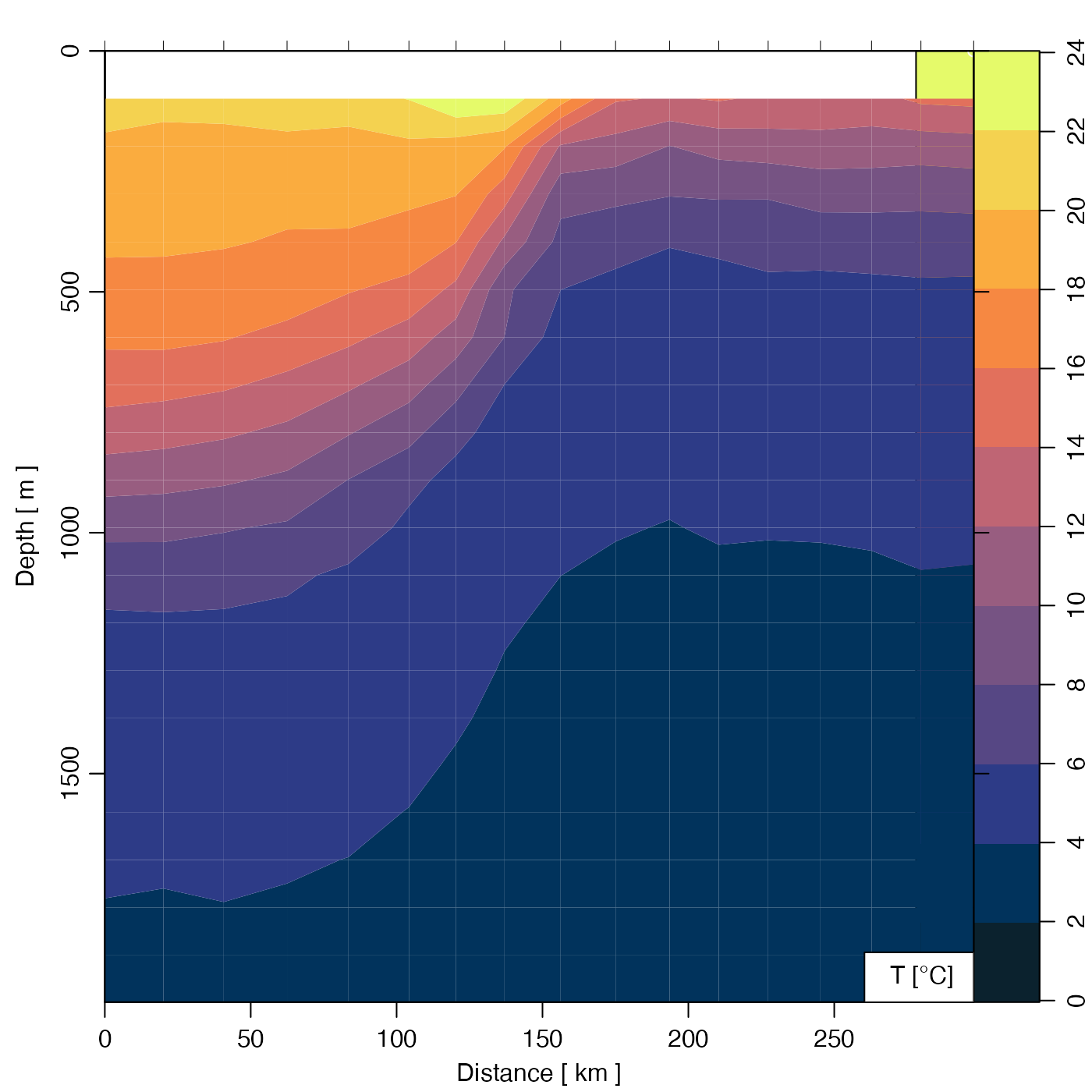Creates a summary plot for a CTD section, with one panel for each value of which.

# S4 method for section
plot(
x,
which = c(1, 2, 3, 99),
eos,
at = NULL,
labels = TRUE,
grid = FALSE,
contourLevels = NULL,
contourLabels = NULL,
stationIndices,
coastline = "best",
xlim = NULL,
ylim = NULL,
zlim = NULL,
zbreaks = NULL,
zcol = NULL,
map.xlim = NULL,
map.ylim = NULL,
clongitude,
clatitude,
span,
projection = NULL,
xtype = "distance",
ytype = "depth",
ztype = "contour",
longitude0,
latitude0,
legend.loc = "bottomright",
legend.text = NULL,
showStations = FALSE,
showStart = TRUE,
stationTicks = TRUE,
showBottom = TRUE,
showSpine = TRUE,
drawPalette = TRUE,
axes = TRUE,
mgp,
mar,
col,
cex,
pch,
labcex = 1,
debug,
...
)

## Arguments

x

a section object.

which

a list of desired plot types, as explained in “Details”. There may be up to four panels in total, and the desired plots are placed in these panels, in reading order. If only one panel is plotted, par is not adjusted, which makes it easy to add to the plot with subsequent plotting commands.

eos

Character indication of the seawater equation of state to use. The permitted choices are "gsw" and "unesco". If eos is not supplied, it defaults to getOption("oceEOS",default="gsw").

at

If NULL (the default), the x axis will indicate the distance of the stations from the first in the section. (This may give errors in the contouring routine, if the stations are not present in a geographical order.) If a list, then it indicates the values at which stations will be plotted.

labels

Either a logical, indicating whether to put labels on the x axis, or a vector that is a list of labels to be placed at the x positions indicated by at.

grid

If TRUE, points are drawn at data locations.

contourLevels

Optional contour levels.

contourLabels

Optional contour labels.

stationIndices

Optional list of the indices of stations to use. Note that an index is not a station number, e.g. to show the first 4 stations, use station.indices=1:4.

coastline

Either a coastline object to be used, or a string. In the second case, the permitted choices are "best" (the default) to pick a variant that suits the scale, "coastlineWorld" for the coarse version that is provided by oce, "coastlineWorldMedium" or "coastlineWorldFine" for two coastlines provided by the ocedata package, or "none", to avoid drawing a coastline.

xlim

Optional limit for x axis (only in sections, not map).

ylim

Optional limit for y axis (only in sections, not map)

zlim, zbreaks, zcol

Elements that control colours for image and points plot types, i.e. if ztype is either "points" or "image". zlim is a two-element numerical vector specifying the limit on the plotted field. If not provided, it defaults to the data range. zbreaks controls the colour breaks, in a manner that is similar to the image() parameter named breaks. If not provided, zbreaks is inferred from zlim. zcol, which controls the colour scheme, may be a vector of colours (of length 1 less than zbreaks), or a function that takes an integer as its sole argument and returns that number of colours. If not provided, zcol defaults to oceColorsViridis(). These three parameters are used in Example 6, an illustration of Atlantic salinity along 36N.

map.xlim, map.ylim

Optional limits for station map; map.ylim is ignored if map.xlim is provided.

clongitude, clatitude, span

Optional map centre position and span (km).

projection

Parameter specifying map projection; see mapPlot(). If projection="automatic", however, a projection is devised from the data, with stereographic if the mean latitude exceeds 70N and mollweide otherwise.

xtype

Type of x axis, for contour plots, either "distance" for distance (in km) to the first point in the section, "track" for distance along the cruise track, "longitude", "latitude", "time" or "spine" (distance along a spine that was added with addSpine()). Note that if the x values are not in order, they will be put in order, and since that might not make physical sense, a warning will be issued.

ytype

Type of y axis for contour plots, either "pressure" for pressure (in dbar, with zero at the surface) or "depth" for depth (in m below the surface, calculated from pressure with swDepth()).

ztype

String indicating whether to how to indicate the "z" data (in the R sense, i.e. this could be salinity, temperature, etc; it does not mean the vertical coordinate) The choices are: "contour" for contours, "image" for an image (drawn with imagep() with filledContours=TRUE), or "points" to draw points. In the first two cases, the data must be gridded, with identical pressures at each station.

longitude0, latitude0

Location of the point from which distance is measured. These values are ignored unless xtype is "distance".

legend.loc

Location of legend, as supplied to legend(), or set to the empty string to avoid plotting a legend.

legend.text

character value indicating the text for the legend. If this is NULL (the default) then the legend is automatically constructed by labelWithUnit(), based on the value of which.

showStations

Logical indicating whether to draw station numbers on maps.

showStart

Logical indicating whether to indicate the first station with

stationTicks

A logical value indicating whether to indicate station locations with ticks at the top margin of cross-section plots. Setting this parameter to FALSE frees the user up to do their own labelling at this spot.

showBottom

a value indicating whether (and how) to indicate the ocean bottom on cross-section views. There are three possibilities. (a) If showBottom is FALSE, then the bottom is not rendered. If it is TRUE, then the bottom is rendered with a gray polygon. (b) If showBottom is the character value "polygon", then a polygon is drawn, and similarly lines are drawn for "lines", and points for "points". (c) If showBottom is a topo object, then the station locations are interpolated to that topography and the results are shown with a polygon. See “Examples”.

showSpine

logical value used if which="map". If showSpine is TRUE and section has had a spine added with addSpine(), then the spine is drawn in blue.

drawPalette

Logical value indicating whether to draw a palette when ztype="image" ignored otherwise.

axes

Logical value indicating whether to draw axes.

mgp

A 3-element numerical vector to use for par(mgp), and also for par(mar), computed from this. If not provided, this defaults to getOption("oceMgp").

mar

Value to be used with par("mar"). If not provided, a default is set up.

col

Color for line types. If not provided, this defaults to par("col"). See zcol, for ztype="image" and ztype="points".

cex

Numerical character-expansion factor, which defaults to par("cex").

pch

Indication of symbol type; defaults to par("pch").

labcex

Size of characters in contour labels (passed to contour()).

debug

an integer specifying whether debugging information is to be printed during the processing. This is a general parameter that is used by many oce functions. Generally, setting debug=0 turns off the printing, while higher values suggest that more information be printed. If debug is not supplied, it defaults to getOption("oceDebug").

...

Optional arguments passed to the contouring function.

## Value

If the original section was gridded, the return value is that section. Otherwise, the gridded section that was constructed for the plot is returned. In both cases, the value is returned silently. The purpose of returning the section is to enable subsequent processing of the grid, including adding elements to the plot (see example 5).

## Details

The type of plot is governed by which, as follows.

• which=0 or "potential temperature" for potential temperature contours

• which=1 or "temperature" for in-situ temperature contours (the default)

• which=2 or "salinity" for salinity contours

• which=3 or "sigmaTheta" for sigma-theta contours

• which=4 or "nitrate" for nitrate concentration contours

• which=5 or "nitrite" for nitrite concentration contours

• which=6 or "oxygen" for oxygen concentration contours

• which=7 or "phosphate" for phosphate concentration contours

• which=8 or "silicate" for silicate concentration contours

• which=9 or "u" for eastward velocity

• which=10 or "uz" for vertical derivative of eastward velocity

• which=11 or "v" for northward velocity

• which=12 or "vz" for vertical derivative of northward velocity

• which=20 or "data" for a dot for each data location

• which=99 or "map" for a location map

The y-axis for the contours is pressure, plotted in the conventional reversed form, so that the water surface appears at the top of the plot. The x-axis is more complicated. If at is not supplied, then the routine calculates x as the distance between the first station in the section and each of the other stations. (This will produce an error if the stations are not ordered geographically, because the contour() routine cannot handle non-increasing axis coordinates.) If at is specified, then it is taken to be the location, in arbitrary units, along the x-axis of labels specified by labels; the way this works is designed to be the same as for axis().

## Ancillary Examples

The following examples were once part of the “Examples” section, but were moved here in May 2022, to reduce the build-check time for CRAN submission.

library(oce)
data(section)
GS <- subset(section, 113<=stationId&stationId<=129)
GSg <- sectionGrid(GS, p=seq(0, 2000, 100))

# Gulf Stream, salinity data and contoured
par(mfrow=c(2, 1))
plot(GS, which=1, ylim=c(2000, 0), ztype="points",
zbreaks=seq(0,30,2), pch=20, cex=3)
plot(GSg, which=1, ztype="image", zbreaks=seq(0,30,2))

# Gulf Stream, temperature grid (image) and data (dots)
par(mfrow=c(1, 1))
plot(GSg, which=1, ztype="image")
T <- GS[["temperature"]]
col <- oceColorsViridis(100)[rescale(T, rlow=1, rhigh=100)]
points(GS[["distance"]],GS[["depth"]],pch=20,cex=3,col="white")
points(GS[["distance"]],GS[["depth"]],pch=20,cex=2.5,col=col)

# 4. Image of temperature, with a high-salinity contour on top;
#    note the Mediterranean water.
sec <- plot(section, which="temperature", ztype="image")
S <- sec[["salinity", "grid:distance-pressure"]]
contour(S$distance, S$pressure, S\$field, level=35.8, lwd=3, add=TRUE)

# 5. Contours of salinity, with dots for high pressure and spice
plot(section, which="salinity")
distance <- section[["distance"]]
depth <- section[["depth"]]
spice <- section[["spice"]]
look <- spice > 1.8 & depth > 500
points(distance[look], depth[look], col="red")

# Image of Absolute Salinity, with 4-minute bathymetry
# It's easy to calculate the desired area for the bathymetry,
# requires the "raster" and "ncdf4" packages to be installed.
if (requireNamespace("raster") && requireNamespace("ncdf4")) {
plot(section, which="SA", xtype="longitude", ztype="image", showBottom=t)
}

# Temperature with salinity added in red
plot(GSg, which="temperature")
distance <- GSg[["distance", "byStation"]]
depth <- GSg[["station", 1]][["depth"]]
S <- matrix(GSg[["salinity"]], byrow=TRUE, nrow=length(GSg[["station"]]))

# Image with controlled colours
plot(GSg, which="salinity", ztype="image",
zlim=c(35, 37.5),
zbreaks=seq(35, 37.5, 0.25),
zcol=oceColorsTurbo)

The documentation for section explains the structure of section objects, and also outlines the other functions dealing with them.

Other functions that plot oce data: download.amsr(), plot,adp-method, plot,adv-method, plot,amsr-method, plot,argo-method, plot,bremen-method, plot,cm-method, plot,coastline-method, plot,ctd-method, plot,gps-method, plot,ladp-method, plot,landsat-method, plot,lisst-method, plot,lobo-method, plot,met-method, plot,odf-method, plot,rsk-method, plot,satellite-method, plot,sealevel-method, plot,tidem-method, plot,topo-method, plot,windrose-method, plot,xbt-method, plotProfile(), plotScan(), plotTS(), tidem-class

Other things related to section data: [[,section-method, [[<-,section-method, as.section(), handleFlags,section-method, initializeFlagScheme,section-method, read.section(), section-class, sectionAddStation(), sectionGrid(), sectionSmooth(), sectionSort(), section, subset,section-method, summary,section-method

Dan Kelley

## Examples

library(oce)
data(section)
GS <- subset(section, 113<=stationId&stationId<=129)
GSg <- sectionGrid(GS, p=seq(0, 2000, 100))

# Gulf Stream, salinity and temperature contours
plot(GSg, which=c("salinity", "temperature"))# Gulf Stream, Temperature image
plot(GSg, which="temperature", ztype="image",
zbreaks=seq(0,25,2), zcol=oceColorsTemperature)